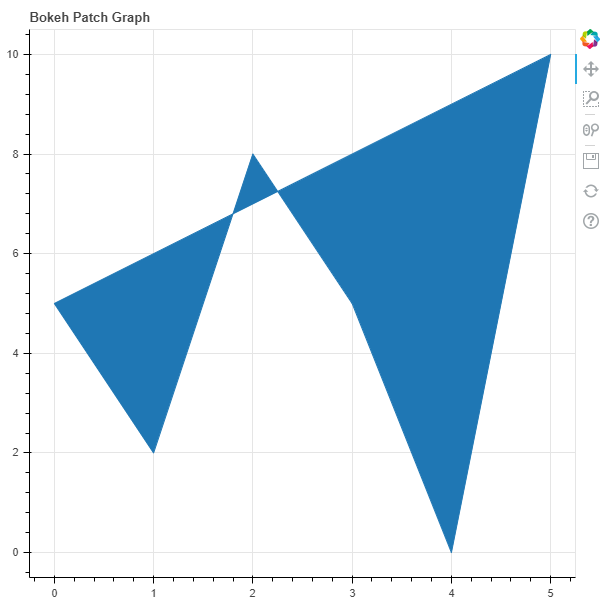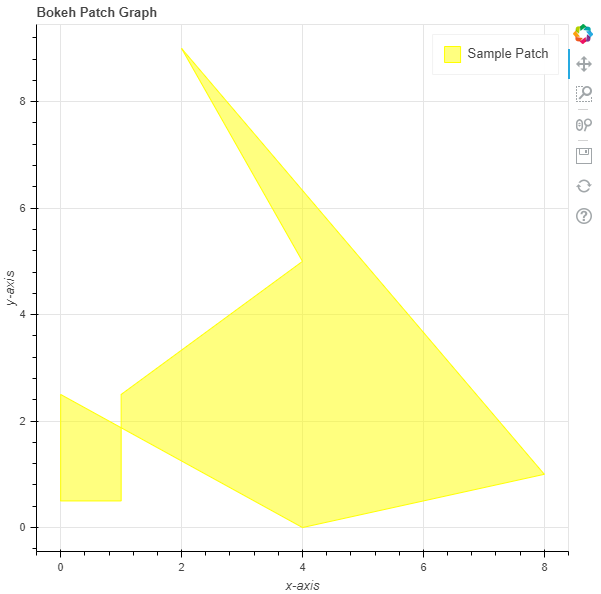Related Articles
Python Bokeh – Plotting Patches on a Graph
• Last Updated : 10 Jul, 2020

Bokeh is a Python interactive data visualization. It renders its plots using HTML and JavaScript. It targets modern web browsers for presentation providing elegant, concise construction of novel graphics with high-performance interactivity.

Bokeh can be used to plot patches on a graph. Plotting patches on a graph can be done using the `patch()` method of the `plotting` module.

## plotting.figure.patch()

Syntax : patch(parameters)

Parameters :

• x : x-coordinates of the patch
• y : y-coordinates of the patch

Returns : an object of class `GlyphRenderer`

Example 1 : In this example we will be using the default values for plotting the graph.

 `# importing the modules ``from` `bokeh.plotting ``import` `figure, output_file, show `` ` `# file to save the model ``output_file(``"gfg.html"``) ``      ` `# instantiating the figure object ``graph ``=` `figure(title ``=` `"Bokeh Patch Graph"``) ``    ` `# the points to be plotted``x ``=` `[``0``, ``1``, ``2``, ``3``, ``4``, ``5``]``y ``=` `[``5``, ``2``, ``8``, ``5``, ``0``, ``10``]``     ` `# plotting the graph ``graph.patch(x, y) ``      ` `# displaying the model ``show(graph)`

Output :Example 2 : In this example we will be plotting the patch with various other parameters

 `# importing the modules ``from` `bokeh.plotting ``import` `figure, output_file, show `` ` `# file to save the model ``output_file(``"gfg.html"``) ``      ` `# instantiating the figure object ``graph ``=` `figure(title ``=` `"Bokeh Patch Graph"``) `` ` `# name of the x-axis ``graph.xaxis.axis_label ``=` `"x-axis"``      ` `# name of the y-axis ``graph.yaxis.axis_label ``=` `"y-axis"`` ` `# points to be plotted``x ``=` `[``0``, ``0``, ``1``, ``1``, ``4``, ``2``, ``8``, ``4``]``y ``=` `[``2.5``, ``0.5``, ``0.5``, ``2.5``, ``5``, ``9``, ``1``, ``0``]`` ` `# color value of the patch``color ``=` `"yellow"`` ` `# fill alpha value of the patch``fill_alpha ``=` `0.5`` ` `# name of the legend``legend_label ``=` `"Sample Patch"`` ` `# plotting the graph ``graph.patch(x, y,``            ``color ``=` `color,``            ``fill_alpha ``=` `fill_alpha,``            ``legend_label ``=` `legend_label) ``      ` `# displaying the model ``show(graph)`

Output :Attention geek! Strengthen your foundations with the Python Programming Foundation Course and learn the basics.

To begin with, your interview preparations Enhance your Data Structures concepts with the Python DS Course.

My Personal Notes arrow_drop_up